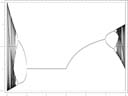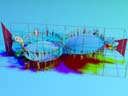# Miscellaneous math trivia that I don't have a good place for:

• ## Bifurcation diagramsHigh-resolution bifurcation diagram of the logistic map xn+1 = rxn(1 - xn) as r goes from -2 to 4.Detail of just the chaotic region from 3.56... to 4, with colors chosen to highlight some of the details.Real parts of logistic map orbit points for complex r. Note that along the real line this corresponds to the standard 2-dimensional bifurcation diagrams shown above. Chaotic filaments other than the ones along the real line have been removed from this diagram for clarity. (The moire patterns seen on the surfaces are artifacts of the algorithm I used to generate this diagram. They shouldn't really be there.)
• ## Rotation matrix for three dimensions

In Cartesian coordinates, the image v' = (vx'vy'vz') of vector v = (vxvyvz) rotated by θ radians in a right-handed direction (counterclockwise when looking toward the origin) about the axis defined by the unit vector a = (axayaz) is given by

v' = Ra(θv,

where

 0 -azθ ayθ ax2 + (1 - ax2) cos θ axay(1 - cos θ) - az sin θ azax(1 - cos θ) + ay sin θ Ra(θ) = exp azθ 0 -axθ = axay(1 - cos θ) + az sin θ ay2 + (1 - ay2) cos θ ayaz(1 - cos θ) - ax sin θ . -ayθ axθ 0 azax(1 - cos θ) - ay sin θ ayaz(1 - cos θ) + ax sin θ az2 + (1 - az2) cos θ

A Mathematica definition for this function is:

```RotationMatrix3D[{ax_, ay_, az_}, theta_] :=
MatrixExp[{{0, -az theta, ay theta}, {az theta, 0, -ax theta},
{-ay theta, ax theta, 0}}]```

or, equivalently,

```RotationMatrix3D[{ax_, ay_, az_}, theta_] :=
{{ax^2 + (1 - ax^2)*Cos[theta], ax*ay*(1 - Cos[theta]) - az*Sin[theta],
az*ax*(1 - Cos[theta]) + ay*Sin[theta]},
{ax*ay*(1 - Cos[theta]) + az*Sin[theta], ay^2 + (1 - ay^2)*Cos[theta],
ay*az*(1 - Cos[theta]) - ax*Sin[theta]},
{az*ax*(1 - Cos[theta]) - ay*Sin[theta],
ay*az*(1 - Cos[theta]) + ax*Sin[theta], az^2 + (1 - az^2)*Cos[theta]}}```